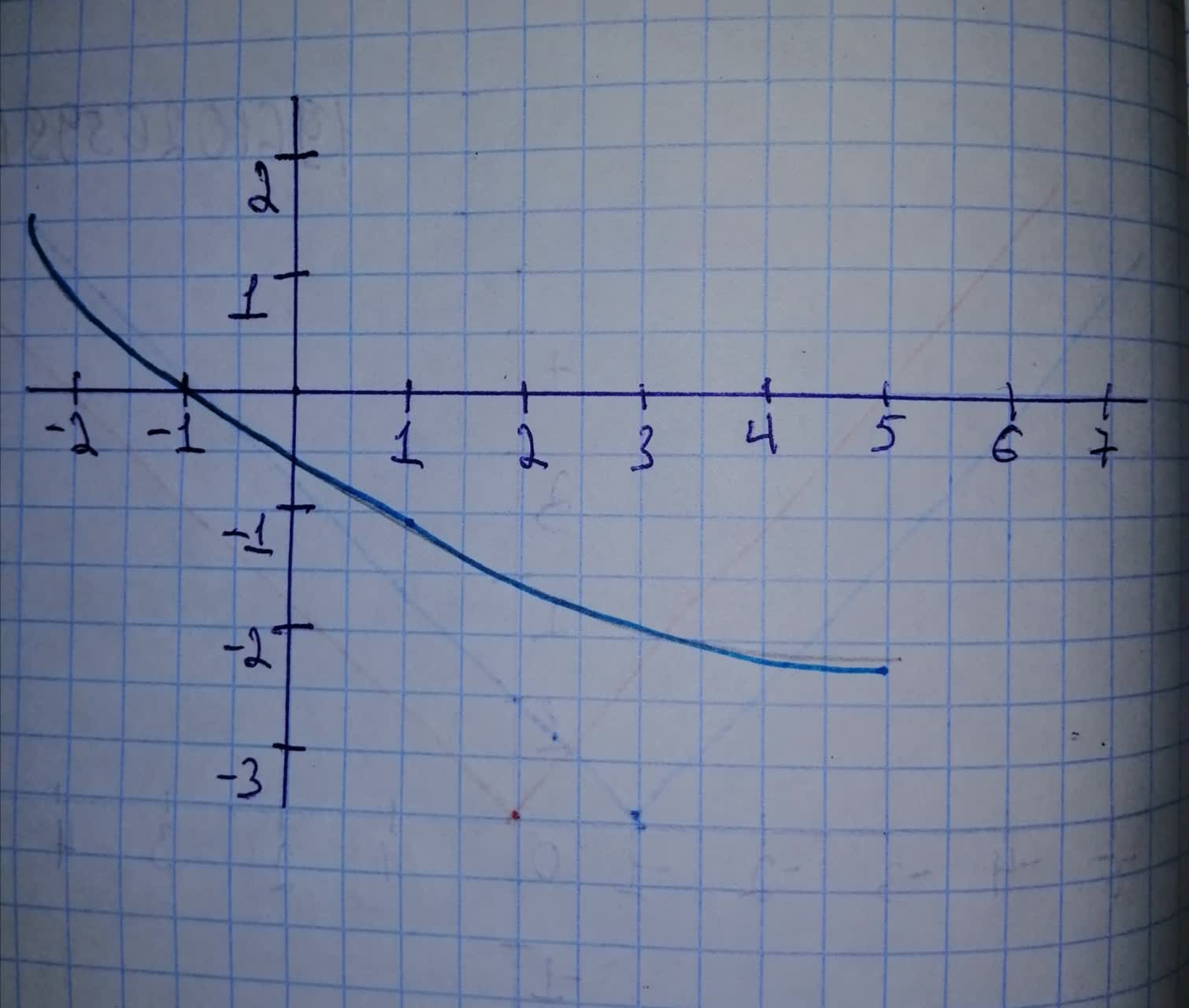Question# Sketch a graph of the function. Use transformations of functions when ever possible. f(x)=1 - sqrt{x + 2}

Transformations of functions
ANSWEREDSketch a graph of the function. Use transformations of functions when ever possible. $$\displaystyle{f{{\left({x}\right)}}}={1}\ -\ \sqrt{{{x}\ +\ {2}}}$$Shown is the graph of $$\displaystyle{f{{\left({x}\right)}}}={1}\ -\ \sqrt{{{x}\ +\ {2}}}$$ x is on the horizontal axis and y is on the vertical axis.